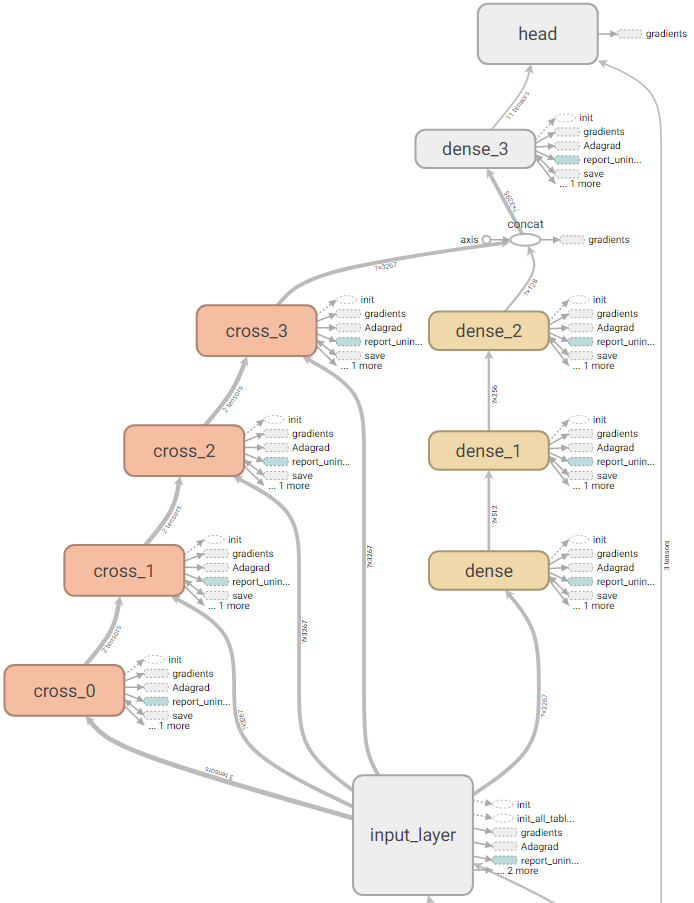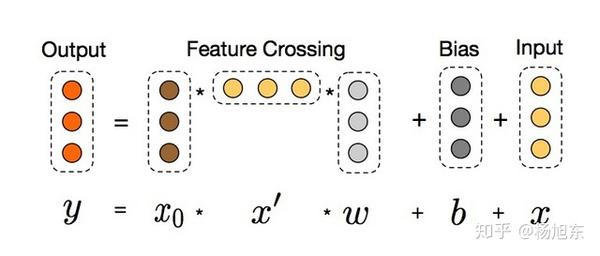Deep & Cross Network(DCN)在 2017 年由 google 和 Stanford 共同发表的一篇论文中被提出，类似于Wide & Deep Network(WDL)，是用复杂网络预估CTR的一种方法。

## DCN网络结构

DCN模型以一个嵌入和堆叠层(embedding and stacking layer)开始，接着并列连一个cross network和一个deep network，接着通过一个combination layer将两个network的输出进行组合。

## 嵌入和堆叠层embed0 = tf.feature_column.embedding_column(...)
...
dense0 = tf.feature_column.indicator_column(...)
dense1 = tf.feature_column.numeric_column(...)
...
columns = [embed0, ..., dense0, dense1, ...]
x0 = tf.feature_column.input_layer(features, feature_columns)

## 交叉网络•是列向量（column vectors），分别表示来自第层和第()层cross layers的输出；
•是第层layer的weight和bias参数。Figure 2: Visualization of a cross layer.

def cross_layer(x0, x, name):
with tf.variable_scope(name):
input_dim = x0.get_shape().as_list()
w = tf.get_variable("weight", [input_dim], initializer=tf.truncated_normal_initializer(stddev=0.01))
b = tf.get_variable("bias", [input_dim], initializer=tf.truncated_normal_initializer(stddev=0.01))
xx0 = tf.expand_dims(x0, -1)  # shape <?, d, 1>
xx = tf.expand_dims(x, -1)  # shape <?, d, 1>
mat = tf.matmul(xx0, xx, transpose_b=True)  # shape <?, d, d>
return tf.tensordot(mat, w, 1) + b + x  # shape <?, d>

def cross_layer2(x0, x, name):
with tf.variable_scope(name):
input_dim = x0.get_shape().as_list()
w = tf.get_variable("weight", [input_dim], initializer=tf.truncated_normal_initializer(stddev=0.01))
b = tf.get_variable("bias", [input_dim], initializer=tf.truncated_normal_initializer(stddev=0.01))
xb = tf.tensordot(tf.reshape(x, [-1, 1, input_dim]), w, 1)
return x0 * xb + b + x

def build_cross_layers(x0, params):
num_layers = params['num_cross_layers']
x = x0
for i in range(num_layers):
x = cross_layer2(x0, x, 'cross_{}'.format(i))
return x

## 深度网络•别是第层和第()层hidden layer；
•个deep layer的参数；
•是ReLU function。

def build_deep_layers(x0, params):
# Build the hidden layers, sized according to the 'hidden_units' param.
net = x0
for units in params['hidden_units']:
net = tf.layers.dense(net, units=units, activation=tf.nn.relu)
return net

## Combination Layerdef dcn_model_fn(features, labels, mode, params):
x0 = tf.feature_column.input_layer(features, params['feature_columns'])
last_deep_layer = build_deep_layers(x0, params)
last_cross_layer = build_cross_layers(x0, params)
last_layer = tf.concat([last_cross_layer, last_deep_layer], 1)
logits = tf.layers.dense(last_layer, units=my_head.logits_dimension)
features=features,
mode=mode,
labels=labels,
logits=logits,
train_op_fn=lambda loss: optimizer.minimize(loss, global_step=tf.train.get_global_step())
)

## 总结

DCN主要有以下几点贡献：

• 提出一种新型的交叉网络结构，可以用来提取交叉组合特征，并不需要人为设计的特征工程；
• 这种网络结构足够简单同时也很有效，可以获得随网络层数增加而增加的多项式阶（polynomial degree）交叉特征；
• 十分节约内存（依赖于正确地实现），并且易于使用；
• 实验结果表明，DCN相比于其他模型有更出色的效果，与DNN模型相比，较少的参数却取得了较好的效果。

## 论文阅读

Deep & Cross Network for Ad Click Prediction